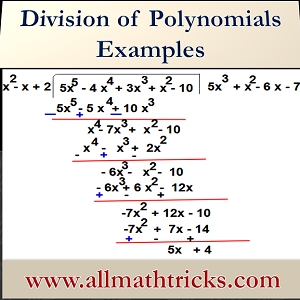# Dividing Polynomials | Division of polynomials examples with solutions

Division of a polynomial by another polynomial is one of the important  concept in Polynomial expressions. In this article explained about  basic phenomena of diving polynomial algorithm in step by step process

## Algebra division| Dividing Polynomials Long Division

Before going to algebra divisions observe the normal numerical division algorithm

When we divide 137 by 5 we get the quotient 27 and remainder 2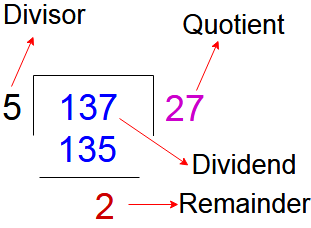We can write 137 as

137 = (5 x 27) + 2   (Note : Here remainder 2 and it is less than divisor 5)

i.e Dividend = Divisor x Quotient + Remainder

The same division algorithm of number is also applicable for division algorithm of polynomials.

i.e When a polynomial divided by another polynomial

Dividend = Divisor x Quotient + Remainder, when remainder is zero or polynomial of degree less than that of divisor

A polynomial f(x) is divided by another polynomial g(x) we get quotient q(x) and remainder p(x) such that

f(x) = g(x) . q(x) + p(x)

Where p(x) = 0 or degree of p(x) < degree of g(x)

### Polynomial long division examples with solution

#### Dividing polynomials by monomials

Take one example

Example -1 :  Divide the polynomial 2x4 +3x2 +x  by x

Here= 2x3 + 3x +1

So we write the polynomial 2x4 +3x2 +x as product of x and 2x3 + 3x +1

2x4 +3x2 +x = (2x3 + 3x +1) x

It means x & 2x3 + 3x +1 are factors of 2x4 +3x2 +x

Example – 2 : Divide the polynomial 3x3 + 9x2 + 5 by 3xHere variable “x” in denominator so it is not a polynomial.

So polynomial of 3x3 + 9x2 + 5 can be written as

3x3 + 9x2 + 5 = 3x (x2 + 3x) +5

Here we can say (x2 + 3x) is the quotient, 3x is the divisor and 5 is the remainder. And also we can say that the reminder is not zero, 3x is not a factor of 3x3 + 9x2 + 5

#### Dividing Polynomials by Binomials

Example – 3: Divide the polynomial 4x2-3x +x3 +10 by x+4 and verify the remainder with zero of the divisor

Solution: Say f(x) = 4x2-3x +x3 +10  and g(x)= x+4

For this division, we have using the steps as follows

Step: 1 – Arrange the terms of the dividend f(x) and the divisor g(x) in descending order of their degree

f(x)= x3 +4x2-3x  +10 and g(x) = x+4

Step:2 – We get first term of quotient by dividing the first term of dividend (i.e  x3 ) with first term of divisor (i.e x)

SoStep:3 – Now the first term of quotient x2 multiplying the divisor g(x) and that term subtract from dividend

x2 (x +4) = x3 +4x2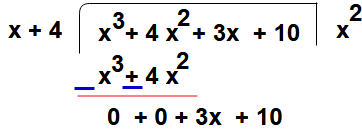Step : 4 –  Now repeat the step 2 again

i.e  To get second term of quotient by dividing the first term of get remainder in previous step (i.e  3x ) with first term of divisor (i.e x)

SoStep:5 – Then second term of quotient 3 multiplying the divisor g(x) and that term subtract from remainder got in step 3

3(x +4) = 3x + 12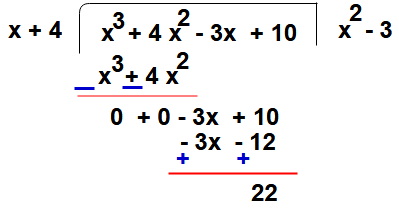Here the quotient is (x2 – 3) and remainder is 22

Here we can write this division process as

Dividend = Divisor x Quotient + Remainder

f(x)  = (x2 – 3) g(x) + 22

x3 +4x2-3x  +10 =  (x2 – 3) ( x+4) + 22

By using the remainder theorem we obtaining the remainder  without performing the above process

Note : Remainder theorem applicable only for divisor have linear polynomial with one variable

Consider zero of the polynomial of g(x) is   – 4

g(x) = x +4

g(-4) = -4 +4 = 0

Now find the value of f(x) at x= -4

f(4) = 4 (-4)2 – 3(-4) + (-4)3 + 10 = 22

#### Dividing Polynomials by Trinomials

Example  -4: Divide the polynomial  5x+ x2  + 3x3 –  4x4– 10  by  2 +  x2 – x

Solution:  Say f(x) = 5x+ x2  + 3x3 –  4x4– 10 and g(x)= 2 +  x2 – x

Now divide f(x) by g(x) by the following steps

Step: 1 – Arrange the terms of the dividend f(x) and the divisor g(x) in descending order of their degree

f(x)= 5x– 4x+ 3x3  + x2 – 10  and g(x) = x2 – x  + 2

Step:2 – We get first term of quotient by dividing the first term of dividend (i.e  5x5 ) with first term of divisor (i.e x2)

SoStep : 3 – Now the first term of quotient 5x3 multiplying the divisor g(x) and that term subtract from dividend

5x3 (x2 – x  + 2) = 5x3 – 5x4 + 10x3Step : 4 –  Now repeat the step 2 again

i.e  To get second term of quotient by dividing the first term of get remainder in previous step (i.e  x4 ) with first term of divisor (i.e x2)

SoStep:5 – Then second term of quotient x2 multiplying the divisor g(x) and that term subtract from remainder got in step 3

x2 (x2 – x  + 2) = x4 – x3  + 2x2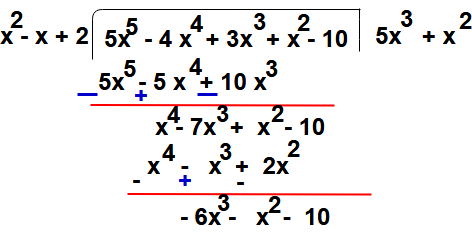Step : 6 –  Now repeat the step 4 again

i.e  To get third term of quotient by dividing the first term of get remainder in previous step (i.e  -6x3 ) with first term of divisor (i.e x2)

SoStep:7 – Then third term of quotient -6x multiplying the divisor g(x) and that term subtract from remainder got in step 5

-6x (x2 – x  + 2) = -6x + 6x2  – 12x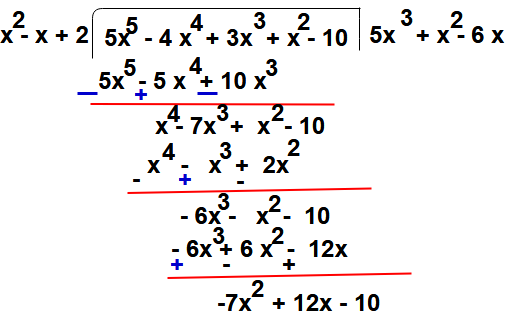Step : 8 –  Now repeat the step 6 again

i.e  To get forth term of quotient by dividing the first term of get remainder in previous step (i.e  -7x2 ) with first term of divisor (i.e x2)

SoStep:9 – Then forth term of quotient -7 multiplying the divisor g(x) and that term subtract from remainder got in step 7

-7 (x2 – x  + 2) = -7x + 7x  – 14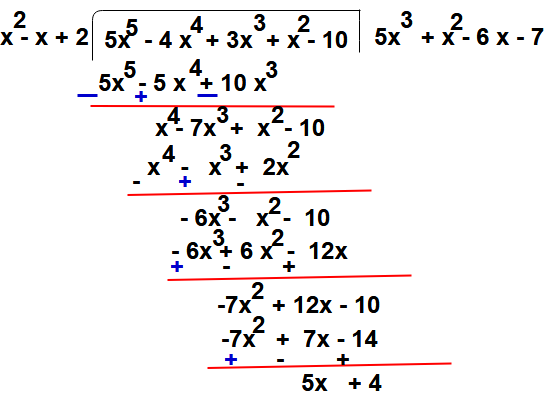Here the division process is completed because degree of (5x + 4)  = 1 which is less than the degree of the divisor (x2 – x  + 2)

So the quotient is (5x3  + x3  -6x – 7) and remainder is 5x + 4

Here we can write this division process as

Dividend = Divisor x Quotient + Remainder

f(x)  =  (5x3  + x3  -6x – 7) g(x) + ( 5x + 4)

(5x+ x2  + 3x3 –  4x4– 10)  =  (5x3  + x3  -6x – 7)  (x2 – x  + 2)  + ( 5x + 4)

Example: Divide the polynomial   1 + x  + 5x-4x4  + 3x3 – 2x2  by  2 + x +  x+ x3

Solution:  Say f(x) = 1 + x  + 5x-4x4  + 3x3 – 2x2  and g(x)= 2 + x +  x+ x3

Now divide f(x) by g(x) by the following steps

Step: 1 – Arrange the terms of the dividend f(x) and the divisor g(x) in descending order of their degree

f(x)= 5x-4x4  + 3x3 – 2x2 + x +1   and g(x) = x3 +  x+ x + 2

Step:2 – We get first term of quotient by dividing the first term of dividend (i.e  5x5 ) with first term of divisor (i.e x3)

SoStep:3 – Now the first term of quotient 5x2 multiplying the divisor g(x) and that term subtract from dividend

5x2 (x3 +  x+ x + 2) = 5x5 + 5x4 + 5x+  10x2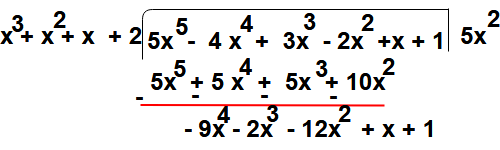Step : 4 –  Now repeat the step 2 again

i.e  To get second term of quotient by dividing the first term of get remainder in previous step (i.e  -9x4 ) with first term of divisor (i.e x3)

SoStep:5 – Then second term of quotient -9x multiplying the divisor g(x) and that term subtract from remainder got in step 3

-9x  (x3 +  x+ x + 2)  = -9x4 – 9x3  – 9x– 18x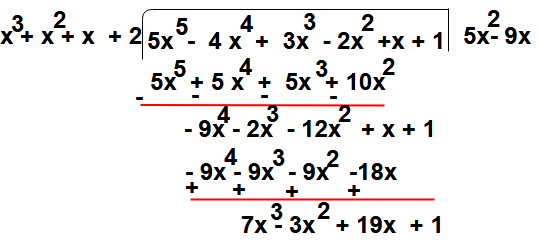Step : 6 –  Now repeat the step 4 again

i.e  To get third term of quotient by dividing the first term of get remainder in previous step (i.e  7x3 ) with first term of divisor (i.e x3)

SoStep:7 – Then third term of quotient  7  multiplying the divisor g(x) and that term subtract from remainder got in step 5

7 (x3 +  x+ x + 2) = 7x3 + 7 x+ 7x + 14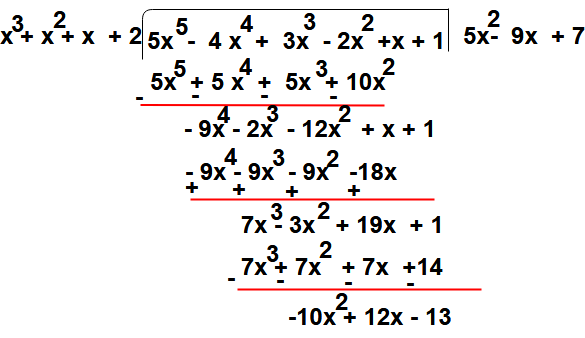Here the division process is completed because degree of (- 10x2 + 12x  -13 )  = 2 which is less than the degree of the divisor (x3 +  x+ x + 2)

So the quotient is (5x2  -9x  + 7) and remainder is  -10x2  + 12x  -13

Here we can write this division process as

Dividend = Divisor x Quotient + Remainder

f(x)  =   (5x2  -9x  + 7) g(x) + ( -10x2  + 12x  -13 )

(5x-4x4  + 3x3 – 2x2 + x +1) =   (5x2  -9x  + 7) (x3 +  x+ x + 2) + ( -10x2  + 12x  -13 )